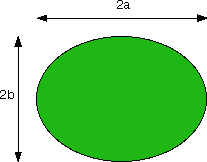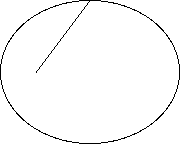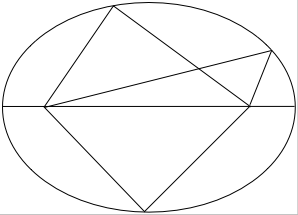There are several potential definitions of an ellipse and it is not trivial to prove that these definitions are equivalent.
1 Affine transformation of a circle.
x2/a2 + y2/b2 = 1
defines an ellipse with width 2a and height 2b centered at the origin.2 Kepler’s equation
r = 1/(1 − e sinθ)
in polar coördinates defines an ellipse with a focus at the origin and eccentricity e.3 String Construction
Another construction is the set of points where the sum of two distances to the two foci is constant.4 Conic Section
Then there is the definition the intersection of a cone and a plane.

No two of these definitions are easily proven to be equivalent. The Dandelin spheres are a fairly easy connection between definitions 3 and 4.